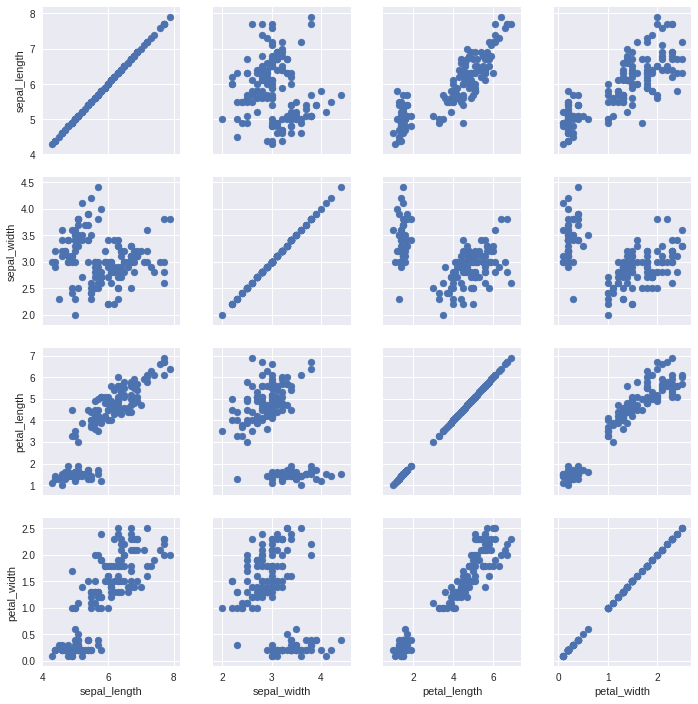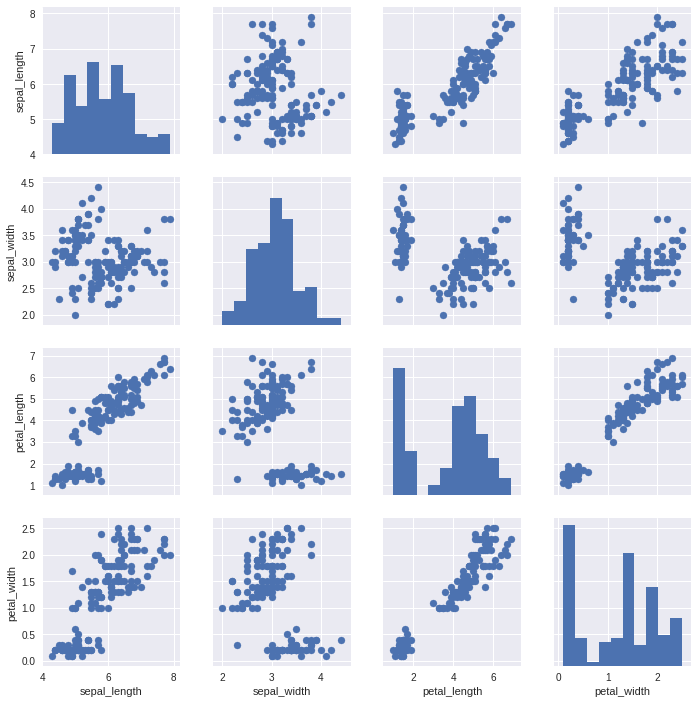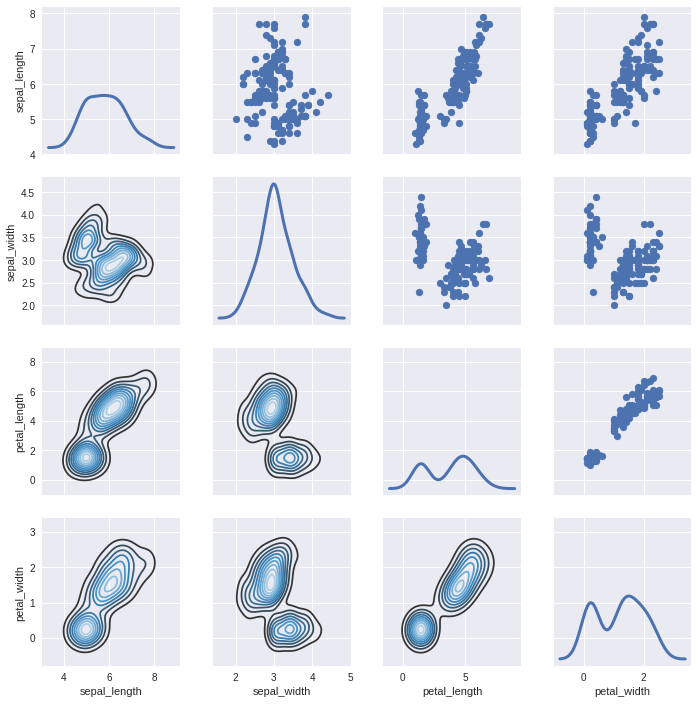# Seaborn - Pair Grid Tutorial¶

PairGrid allows us to draw a grid of subplots using the same plot type to visualize data.

Unlike FacetGrid, it uses a different pairs of a variable for each subplot. It forms a matrix of sub-plots. It is also sometimes called a “scatterplot matrix”.

The usage of pairgrid is similar to facetgrid. First, initialize the grid and then pass the plotting function.

In :
import pandas as pd
import seaborn as sb
from matplotlib import pyplot as plt
g = sb.PairGrid(df)
g.map(plt.scatter);
plt.show()It is also possible to plot a different function on the diagonal to show the univariate distribution of the variable in each column.

In :
import pandas as pd
import seaborn as sb
from matplotlib import pyplot as plt
g = sb.PairGrid(df)
g.map_diag(plt.hist)
g.map_offdiag(plt.scatter);
plt.show()We can customize the color of these plots using another categorical variable. For example, the iris dataset has four measurements for each of three different species of iris flowers so you can see how they differ.

In :
import pandas as pd
import seaborn as sb
from matplotlib import pyplot as plt
g = sb.PairGrid(df)
g.map_diag(plt.hist)
g.map_offdiag(plt.scatter);
plt.show()In :
import pandas as pd
import seaborn as sb
from matplotlib import pyplot as pltDolly Solanki